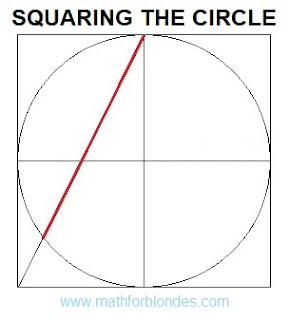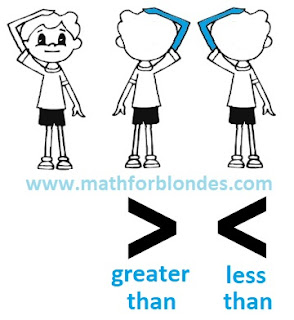## 1.29.2022

### Squaring the circle

Squaring the circle. The solution of the problem. Almost accurate. Math draw.Squaring the circle. The solution of the problem. Almost accurate.

This construction is not a solution to the problem of squaring the circle, but it forced me to get my hands on the math and do all the verification calculations. If the correct solution should give a segment with a length of 1.77245 radii, then the length of the red segment in the figure is 1.78885 radii. A little more than necessary. How much more? The segment exceeds the required length by 0.9253% or by 0.01640 of the radius. The area of a square with sides equal to the length of this segment is 1.8592% greater than the area of a circle.

How to use it? For a friendly prank of math lovers. Build a square with sides equal to ten centimeters. Inscribe in a square a circle with a radius of 5 centimeters. Draw a line as shown in the figure and offer a ruler to measure the length of the resulting segment. It will be equal to 8.9 centimeters. Using a calculator, calculate the area of a circle, which is equal to 78.54 square centimeters. The area of a square with sides of 8.9 centimeters is 79.21 square centimeters. This discrepancy can be explained by the low accuracy of measurements using a ruler. Personally, after such rough calculations, I sat down to check the solution.

I don't recommend this kind of joke with my teachers or math teachers - they can force you to do all the verification calculations to refute this solution. The unsolvability of this problem was proved by the German mathematician Lindemann back in 1882. However, I have long been very distrustful of many proofs of mathematicians.

## 1.01.2022

### Signs greater than and less than

How to remember signs greater than and less than? A very long time ago, when I was little and went to school, the teacher taught us this. Even as an adult, I have always used this method.

We always do more with our right hand than with our left. If we bend our right arm at the elbow and raise it above our head, we get the "GREATER THAN" sign. We do less with our left hand. A bent and raised left hand will give us the "LESS THAN" sign. The elbow of the right hand points to the direction of the "GREATER THAN" sign. This is how it looks in the picture.Signs greater than and less than

Indeed, the arm bent at the elbow is very similar to the "greater than" and "less than" symbols. You don't even have to raise your hand. And this cheat sheet will always be with you. If mathematicians don't cut your hands off.

If you are left-handed and do more with your left hand than with your right? How then to be? You have two options. Or you think like everyone else and you won't have any problems with math. Or you write your "left-handed math" and learn a lot of interesting things.